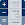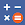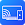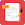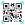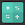# Basic Calculator: GPA & Math

by Apero Technologies Group - TrustedApp
5,000,000+ installs

Android application Basic Calculator: GPA & Math developed by is listed under category Productivity. According to Google Play Basic Calculator: GPA & Math achieved more than 5,000,000 installs. Basic Calculator: GPA & Math currently has 91,054 ratings with average rating value of 4.67. The current percentage of ratings achieved in last 30 days is 5.57%, percentage of ratings achieved in last 60 days is 11.04%. Basic Calculator: GPA & Math has the current market position #9928 by number of ratings. A sample of the market history data for Basic Calculator: GPA & Math can be found below. Last update on 2023-01-17.

Title: Basic Calculator: GPA & Math Apero Technologies Group - TrustedApp Productivity Free Android
Total ratings: 91054 5.57% 11.04% 4.67
Installs (achieved): 5,000,000+ 9,992,318
5 star ratings: 79,020 5,013 1,002 1,403 4,612

2022-04-01: Android application Basic Calculator: GPA & Math achieved 5,000,000 installs. Android application Basic Calculator: GPA & Math achieved 1,000,000 installs. Android application Basic Calculator: GPA & Math achieved 500,000 installs. Android application Basic Calculator: GPA & Math achieved 100,000 installs.

Total number of ratings
Total number of active users rated for Basic Calculator: GPA & Math.

Total number of installs (*estimated)
Estimation of total number of installs on Google Play. Approximated from number of ratings and install bounds achieved on Google Play.

Average rating
Average rating value on Google Play. Given by active users of the application.